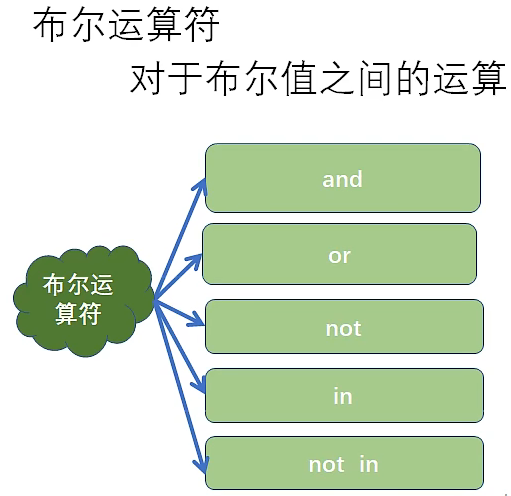### Python运算符——布尔运算符and：逻辑与，并且

``a,b=1,2print("-----------and 并且-------")print(a==1 and b==2)print(a!=1 and b==2)print(a==1 and b<2)``

or：逻辑或，或者

``c,d=10,20print('-------or 或者----------')print(c==10 or d!=20)print(c==10 or d==20)print(c!=10 or d!=20)``

not：对逻辑结果取反

``m=Truen=Falseprint(not m)#not True  ->  Falseprint(not n)#not False ->  True``

in / not in：判断你要的序列是否在指定的序列中，如果是返回True，不是则返回False

``print('--------in /not in------')s='helloworld'print('h' in s)#Trueprint('l' in s)#Trueprint('mm' not in s)#Trueprint('H' in s)#False``